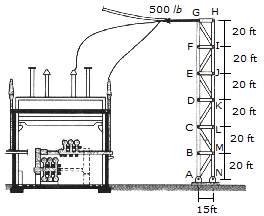### Create an Account

Home / Questions / A tower used in an electrical substation supports a power line which exerts a horizontal ...

# A tower used in an electrical substation supports a power line which exerts a horizontal tension of T 500 lb on each side truss of the tower as shown Determine the force in membersA tower used in an electrical substation supports a power line which exerts a horizontal tension of T = 500 lb on each side truss of the tower as shown. Determine the force in members BC, CM, and LM of a side truss and indicate whether the members are in tension or compression.

 A. CM = 833 lb T, CB = 3330 lb C, LM = 2670 lb T B. CM = 581 lb T, CB = 2050 lb C, LM = 2000 lb T C. CM = 325 lb T, CB = 3040 lb C, LM = 2670 lb T D. CM = 58 lb T, CB = 1646 lb C, LM = 1600 lb T

May 19 2020 View more View LessSubscribe To Get Solution